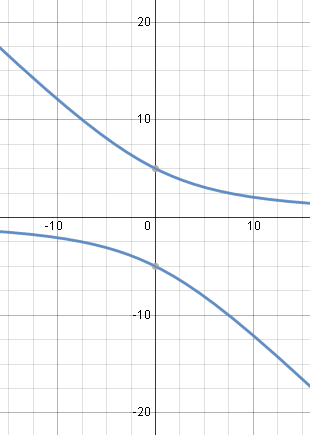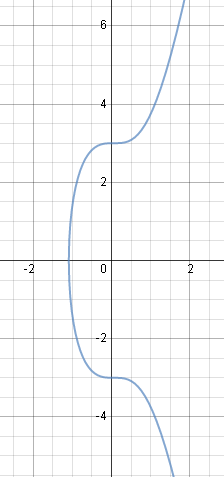Search IntMath
Close

450+ Math Lessons written by Math Professors and Teachers

5 Million+ Students Helped Each Year

1200+ Articles Written by Math Educators and Enthusiasts

Simplifying and Teaching Math for Over 23 Years

# 3. Integrable Combinations

There are many differential equations where we cannot separate the variables, like we saw in the previous section.

However, we can possibly solve the DE if we use one of the following expressions to get the differential equation in a form that we can solve:

(1) d(xy) = x dy + y dx

(2) d(x^2+ y^2) = 2(x dx + y dy)

(3) d(y/x)=(x dy-y dx)/x^2

(4) d(x/y)=(y dx-x dy)/y^2

These differential forms are called integrable combinations. If we can transform our differential equation into one side of one of the above forms, then we can use the other side to solve the DE.

Where do these integrable combinations come from?

One way to think of the first expression above is as follows. Say x and y are both functions of t. If we have the product xy, then the derivative with respect to t of that product would be given by:

d/(dt)(xy)=x(dy)/(dt)+y(dx)/(dt)

(This is just the product rule that we learned ages ago.) Then we can multiply throughout by dt and we get the expression in (1) above, d(xy) = x dy + y dx.

We saw the second combination, d(x^2+ y^2) = 2(x dx + y dy), back in implicit differentiation and related rates.

The third and fourth combinations are based on the Quotient Rule.

### Example 1

Solve

(2y + x)dy + y dx = 0

First, we note that we cannot separate the variables such that the x expressions would be with dx only and the y expressions would be with dy only. So the DE is not separable.

So we aim to get the DE in one of the integrable combination forms. Rearranging, we have:

2y dy + (x dy + y dx) = 0

From the integrable combination (1) given above, the expression in brackets can be written as d(xy) and the differential equation is equivalent to:

2y dy + d(xy) = 0

Integrating gives us:

y2 + xy = K (General Solution)

CHECK

We obtained the solution y2 + xy = K. Is it correct?

Now

d/dx y^2=2y dy/dx (using Implicit Differentiation),

d/dx xy=x dy/dx + y (using product rule).

and d/dx(k)=0.

So putting this together gives the derivative of our answer as:

2y(dy)/(dx)+x(dy)/(dx)+y=0

On multiplying by dx throughout, we have:

2y dy + x dy + y dx = 0

which is the DE that we started with so we know our general solution is correct.

Here is the solution graph of our answer for Example 1 (I've used K = 25) . It is a hyperbola, with asymptotes y = −x, and the x-axis:Solution using K=25.

### Example 2

Solve

x dx = 9x2 dx y dy

We recognise that we can get it in the form of the second expression at the top of this page.

We add y dy to both sides:

x dx + y dy = 9x2 dx

Now multiply both sides by 2:

2(x dx + y dy) = 18x2 dx

We see that the LHS is in the form of the second expression above. Now integrate both sides:

x2 + y2 = 6x3 + K

CHECK

Implicit differentiation gives us:

2x + 2y(dy/dx) = 18x^2

Multiplying throughout by dx/2 gives:

x\ dx + y\ dy = 9x^2dx

Subtracting y dy gives us our original DE

x dx = 9x2 dx y dy

Here is the graph of our answer for Example 2 (using K = 9). It is an implicit function:Solution using K=2.

## Problem SolverThis tool combines the power of mathematical computation engine that excels at solving mathematical formulas with the power of GPT large language models to parse and generate natural language. This creates math problem solver thats more accurate than ChatGPT, more flexible than a calculator, and faster answers than a human tutor. Learn More.